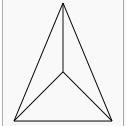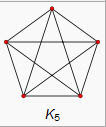## Sunday, 27 December 2009Here are two interesting problems, the second is a little more abstract, but bare with me... there is a purpose...

Suppose there are n parking spaces in a row along a one way street, and n people are driving into town, each with a most preferred spot in the row of spaces. Each of the n people adopts the plan that they will drive along the line until they come to their preferred space. If it is open, they take it, and if not, they continue driving and will take the next available spot. For instance if n=2 parking spaces, then the two drivers would find a parking space if both wanted the first spot, or they both wanted different spots; but one of them is out of luck if they both preferred the last spot, since the one who got there second is out of parking places. Thus for n=2, there are 3 possible solutions out of 2^2 = 4 possible situations. So here are the easy questions, find the number of possible solutions for n=3 and 4, and their associated probabilities of success.
And for the clever people, generalize for n.

If you found that either too hard, too easy, or just want to read on, here is a second problem. For those who have never studied graph theory, or don't remember the terms, a brief introduction. A complete graph with n nodes is called the k_n graph. For n = 4 and 5 the complete graphs look like the ones shown below.Now a spanning Tree is a graph with N vertices but no complete circuits. You can get from any vertex to any other, but only by one path. Here is an example of a tree with six labeled vertices.Finally, we can give the question... For a complete graph of 3 vertices, 4 vertices, or n vertices, how many different spanning trees are possible?

The amazing result is that the two problems seem to be exactly the same, at least they produce the same sequence. The number of solutions for the parking problem is 1, 3, 16, 125, ... (N+1)(n-1)... For the spanning trees, if we start with a graph of 2 points, there is only one tree. For three points there can be three different trees, and for four... yeah..16 . The image below shows all 16 of them as depicted by Wolfram Mathworld.. the sequence is the same but the index is off by one, so we get 1, 3, 16, 125, ... (n)(n-2)... Ok, here is the real question for this blog... why are they the same...Ok, one more curiously beautiful detail about this problem.... the probability of success for the n parking cars at the top has a limit of e/(n+1) as n approaches infinity. I love how often e pops up in these "sorting" type problems..